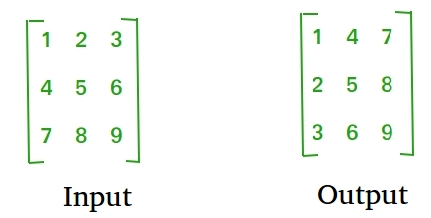GeeksforGeeks App
Open AppBrowser
Continue

# C++ Program To Find Transpose of a Matrix

Transpose of a matrix is obtained by changing rows to columns and columns to rows. In other words, transpose of A[][] is obtained by changing A[i][j] to A[j][i].For Square Matrix :

The below program finds transpose of A[][] and stores the result in B[][], we can change N for different dimension.

## C++

 `// C++ Program to find the transpose``// of a matrix``#include ``using` `namespace` `std;``#define N 4` `// This function stores transpose``// of A[][] in B[][]``void` `transpose(``int` `A[][N],``               ``int` `B[][N])``{``    ``int` `i, j;``    ``for` `(i = 0; i < N; i++)``        ``for` `(j = 0; j < N; j++)``            ``B[i][j] = A[j][i];``}` `// Driver code``int` `main()``{``    ``int` `A[N][N] = {{1, 1, 1, 1},``                   ``{2, 2, 2, 2},``                   ``{3, 3, 3, 3},``                   ``{4, 4, 4, 4}};` `    ``int` `B[N][N], i, j;` `    ``transpose(A, B);` `    ``cout << ``"Result matrix is "``;``    ``for` `(i = 0; i < N; i++)``    ``{``        ``for` `(j = 0; j < N; j++)``           ``cout << ``" "` `<< B[i][j];``        ``cout <<``""``;``    ``}``    ``return` `0;``}`

Output:

```Result matrix is
1 2 3 4
1 2 3 4
1 2 3 4
1 2 3 4```

Time Complexity: O(N*N) as two nested loops are running.

Space Complexity: O(N*N) as 2d array is created to store transpose.

For Rectangular Matrix :

The below program finds transpose of A[][] and stores the result in B[][].

## C++

 `// C++ program to find transpose``// of a matrix``#include ``using` `namespace` `std;``#define M 3``#define N 4` `// This function stores transpose``// of A[][] in B[][]``void` `transpose(``int` `A[][N], ``int` `B[][M])``{``    ``int` `i, j;``    ``for``(i = 0; i < N; i++)``        ``for``(j = 0; j < M; j++)``            ``B[i][j] = A[j][i];``}` `// Driver code``int` `main()``{``    ``int` `A[M][N] = {{1, 1, 1, 1},``                   ``{2, 2, 2, 2},``                   ``{3, 3, 3, 3}};` `    ``// Note dimensions of B[][]``    ``int` `B[N][M], i, j;` `    ``transpose(A, B);` `    ``cout << ``"Result matrix is "``;``    ``for``(i = 0; i < N; i++)``    ``{``        ``for``(j = 0; j < M; j++)``            ``cout << ``" "` `<< B[i][j];``            ` `        ``cout << ``""``;``    ``}``    ``return` `0;``}`

Output:

```Result matrix is
1 2 3
1 2 3
1 2 3
1 2 3```

Time Complexity: O(N*M) as two nested loops are running.

Space Complexity: O(N*M) as 2d array is created to store transpose.

In-Place for Square Matrix:

## C++

 `// C++ program to implement``// the above approach``#include ``using` `namespace` `std;` `#define N 4` `// Converts A[][] to its transpose``void` `transpose(``int` `A[][N])``{``    ``for` `(``int` `i = 0; i < N; i++)``        ``for` `(``int` `j = i+1; j < N; j++)``            ``swap(A[i][j], A[j][i]);``}` `// Driver code``int` `main()``{``    ``int` `A[N][N] = {{1, 1, 1, 1},``                   ``{2, 2, 2, 2},``                   ``{3, 3, 3, 3},``                   ``{4, 4, 4, 4}};` `    ``transpose(A);` `    ``printf``(``"Modified matrix is "``);``    ``for` `(``int` `i = 0; i < N; i++)``    ``{``        ``for` `(``int` `j = 0; j < N; j++)``           ``printf``(``"%d "``, A[i][j]);``        ``printf``(``""``);``    ``}` `    ``return` `0;``}`

Output:

```Modified matrix is
1 2 3 4
1 2 3 4
1 2 3 4
1 2 3 4```

Time complexity: O(n)

• Transpose has a time complexity of O(n + m), where n is the number of columns and m is the number of non-zero elements in the matrix.
• The computational time for transpose of a matrix using identity matrix as reference matrix is O(m*n).
• Suppose, if the given matrix is a square matrix, the running time will be O(n2).

Auxiliary space: O(1).

My Personal Notes arrow_drop_up